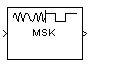# MSK Demodulator Baseband

Demodulate differentially encoded MSK-modulated data

## Library

CPM, in Digital Baseband sublibrary of Modulation

•## Description

The MSK Demodulator Baseband block demodulates a signal that was modulated using the differentially encoded minimum shift keying method. The block expects the input signal to be a baseband representation of a coherent modulated signal with no precoding. The Phase offset parameter represents the initial phase of the modulated waveform.

### Pulse Shape Filtering

Differentially encoded minimum shift keying modulation uses pulse shaping to smooth the phase transitions of the modulated signal. The function q(t) is the phase response obtained from the frequency pulse, g(t), through this relation:$q\left(t\right)={\int }_{-\text{\hspace{0.17em}}\infty }^{t}g\left(t\right)dt$.

The specified frequency pulse shape corresponds to this rectangular pulse shape expression for g(t).

Pulse ShapeExpression
`Rectangular`$g\left(t\right)=\left\{\begin{array}{cc}\frac{1}{2LT},& 0\le t\le LT\\ 0& \text{otherwise}\end{array}$

• L is the main lobe pulse duration in symbol intervals.

• The duration of the pulse, LT, is the pulse length in symbol intervals.

### Integer-Valued Signals and Binary-Valued Signals

This block accepts a scalar-valued or column vector input signal with a data type of `single` or `double`. If you set the Output type parameter to `Integer`, then the block produces values of 1 and -1. If you set the Output type parameter to `Bit`, then the block produces values of 0 and 1.

### Single-Rate Processing

In single-rate processing mode, the input and output signals have the same port sample time. The block implicitly implements the rate change by making a size change at the output when compared to the input. The input width must be an integer multiple of the Samples per symbol parameter value, and the input can be a column vector.

• When you set Output type to `Bit`, the output width is K times the number of input symbols.

• When you set Output type to `Integer`, the output width is the number of input symbols.

### Multirate Processing

In multirate processing mode, the input and output signals have different port sample times. The input must be a scalar. The output symbol time is the product of the input sample time and the Samples per symbol parameter value.

• When you set Output type to `Bit`, the output width equals the number of bits per symbol.

• When you set Output type to `Integer`, the output is a scalar.

### Traceback Depth and Output Delays

Internally, this block creates a trellis description of the modulation scheme and uses the Viterbi algorithm. The Traceback depth parameter, D, in this block is the number of trellis branches used to construct each traceback path. D influences the output delay, which is the number of zero symbols that precede the first meaningful demodulated value in the output.

• When you set the Rate options parameter to `Allow multirate processing`, and the model uses a variable-step solver or a fixed-step solver with the Tasking Mode parameter set to `SingleTasking`, then the delay consists of D+1 zero symbols.

• When you set the Rate options parameter to `Enforce single-rate processing`, then the delay consists of D zero symbols.

The optimal Traceback depth parameter value is dependent on minimum squared Euclidean distance calculations. Alternatively, a typical value, dependent on the number of states, can be chosen using the “five-times-the-constraint-length” rule, which corresponds to 5×log2(numStates). The number of states is determined by the following equation:

$numStates=\left\{\begin{array}{l}p\cdot {2}^{\left(L-1\right)},\text{\hspace{0.17em}}for\text{\hspace{0.17em}}even\text{\hspace{0.17em}}m\\ 2p\cdot {2}^{\left(L-1\right)},\text{\hspace{0.17em}}for\text{\hspace{0.17em}}odd\text{\hspace{0.17em}}m\end{array}\right\}$

where:

• h = m/p is the modulation index proper rational form

• m = numerator of modulation index

• p = denominator of modulation index

• L is the Pulse length

## Parameters

Output type

Determines whether the output consists of bipolar or binary values.

The initial phase of the modulated waveform.

Samples per symbol

The number of input samples that represent each modulated symbol, which must be a positive integer. For more information, see Signal Upsampling and Rate Changes.

Rate options

Select the rate processing method for the block.

• `Enforce single-rate processing` — When you select this option, the input and output signals have the same port sample time. The block implements the rate change by making a size change at the output when compared to the input. The output width is the number of symbols (which is given by dividing the input length by the Samples per symbol parameter value when the Output type parameter is set to `Integer`).

• `Allow multirate processing` — When you select this option, the input and output signals have different port sample times. The output period is the same as the symbol period and equals the product of the input period and the Samples per symbol parameter value.

For more information, see Single-Rate Processing and Multirate Processing in the Description section of this page.

Traceback depth

The number of trellis branches that the MSK Demodulator Baseband block uses to construct each traceback path.

Output data type

The output data type can be `boolean`, `int8`, `int16`, `int32`, or `double`.

## Supported Data Types

PortSupported Data Types

Input

• Double-precision floating point

• Single-precision floating point

Output

• Double-precision floating point

• Boolean (When Output type set to `Bit`)

• 8-, 16-, and 32-bit signed integers (When Output type set to `Integer`)

## Pair Block

MSK Modulator Baseband

## References

 Anderson, John B., Tor Aulin, and Carl-Erik Sundberg, Digital Phase Modulation, New York, Plenum Press, 1986.

## Version History

Introduced before R2006a